## Hyperbolic Geometry

A Non-Euclidean Geometry, also called Lobachevsky-Bolyai-Gauss Geometry, having constant Sectional Curvature. This Geometry satisfies all of Euclid's Postulates except the Parallel Postulate, which is modified to read: For any infinite straight Lineand any Pointnot on it, there are many other infinitely extending straight Lines that pass throughand which do not intersect.

In hyperbolic geometry, the sum of Angles of a Triangle is less than 180°, and Triangles with the same angles have the same areas. Furthermore, not all Triangles have the same Angle sum (c.f. the AAA Theorem for Triangles in Euclidean 2-space). The best-known example of a hyperbolic space are Spheres in Lorentzian 4-space. The Poincaré Hyperbolic Disk is a hyperbolic 2-space. Hyperbolic geometry is well understood in 2-D, but not in 3-D.

Geometric models of hyperbolic geometry include the Klein-Beltrami Model, which consists of an Open Disk in the Euclidean plane whose open chords correspond to hyperbolic lines. A 2-D model is the Poincaré Hyperbolic Disk. Felix Kleinconstructed an analytic hyperbolic geometry in 1870 in which a Point is represented by a pair of Real Numbers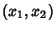with(i.e., points of an Open Disk in the Complex Plane) and the distance between two points is given byThe geometry generated by this formula satisfies all of Euclid's Postulates except the fifth. The Metric of this geometry is given by the Cayley-Klein-Hilbert Metric,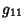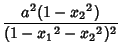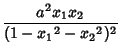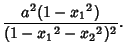Hilbertextended the definition to general bounded sets in a Euclidean Space.

See also Elliptic Geometry, Euclidean Geometry, Hyperbolic Metric, Klein-Beltrami Model, Non-Euclidean Geometry, Schwarz-Pick Lemma

References

Dunham, W. Journey Through Genius: The Great Theorems of Mathematics. New York: Wiley, pp. 57-60, 1990.

Eppstein, D. Hyperbolic Geometry.'' http://www.ics.uci.edu/~eppstein/junkyard/hyper.html.

Stillwell, J. Sources of Hyperbolic Geometry. Providence, RI: Amer. Math. Soc., 1996.